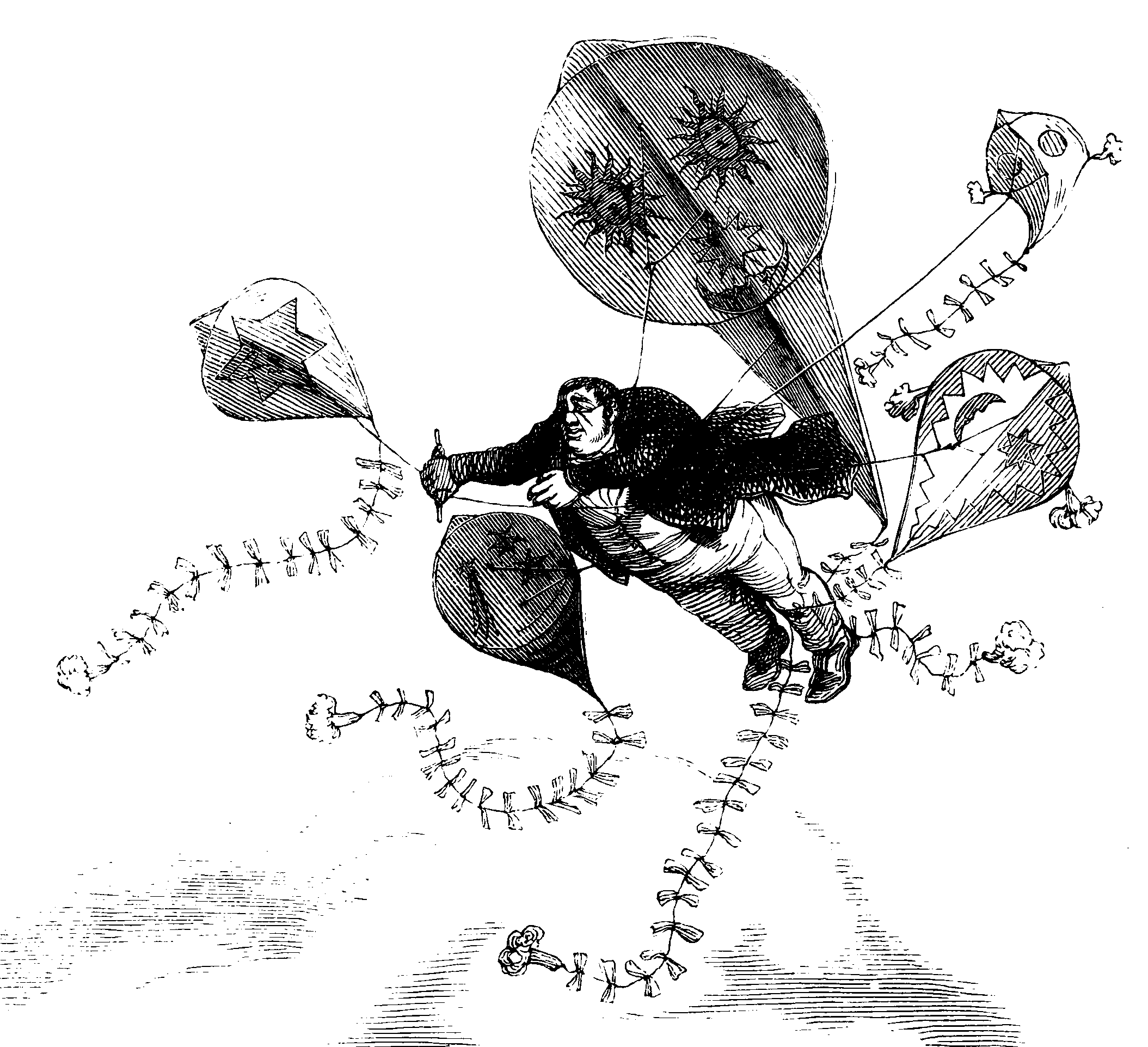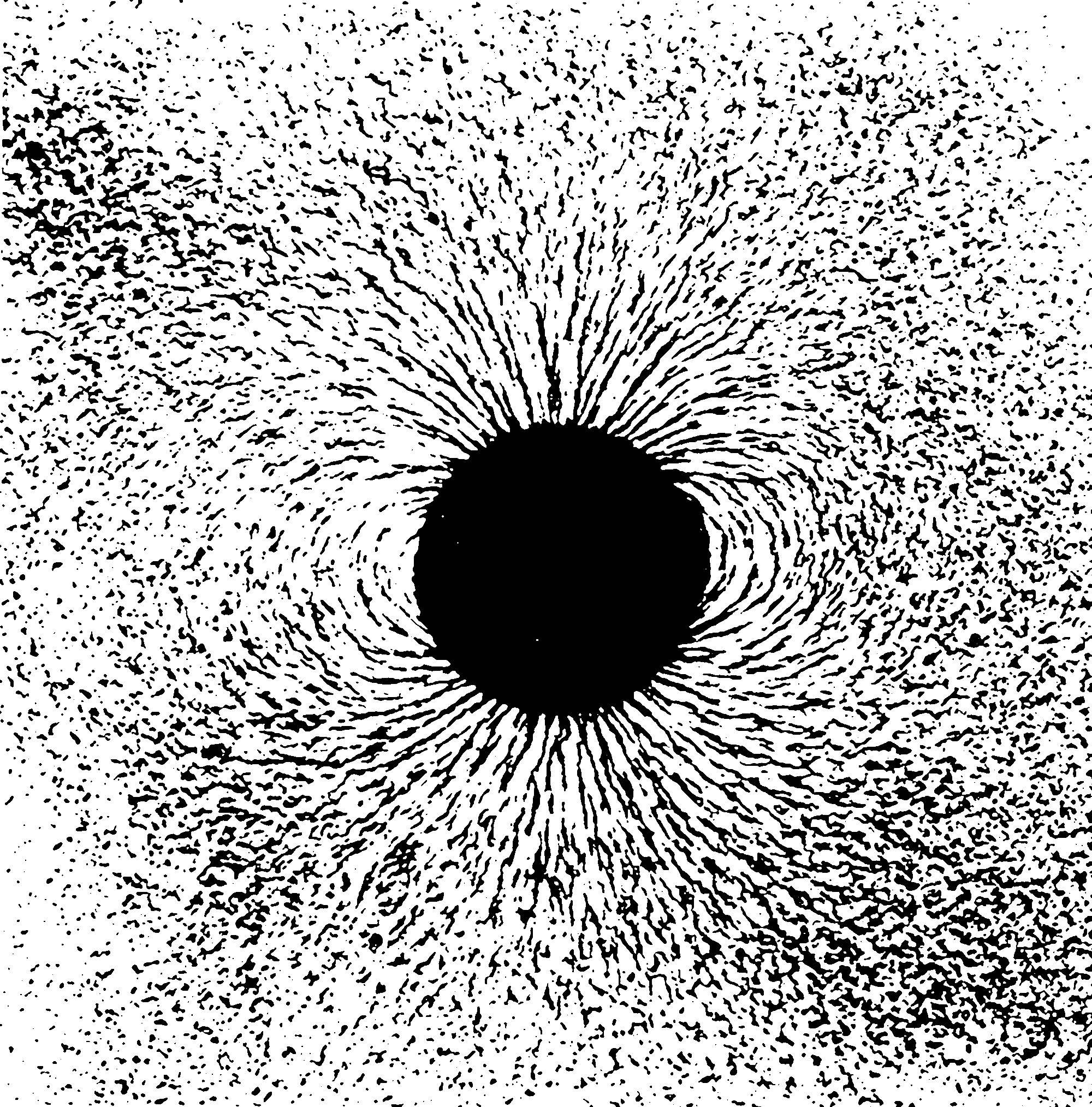# Continuous and equilibrium probabilistic graphical modelsPlaceholder for my notes on probabilistic graphical models over a continuum, i.e. with possibly-uncountably many nodes in the graph; or put another way, where the random field has an uncountable index set (but some kind of structure — a metric space, say.) There is much formalising to be done, which I do not propose to attempt right now, in lieu, here are some notes.

Normally when we discuss graphical models it is in terms of a finitely indexed set of rvs $$\{X_i;i=1,\ldots,n\}$$. If that index $$i$$ ranges instead over a continuum then what does the gaphical model formalism look like?

Here’s a concrete example. Consider Gaussian process whose covariance kernel $$K$$ is continuous and smoothly decays. Let it be over index space $$\mathcal{T}:=\mathbb{R}^n$$ for the sake of argument. It implicitly defines an undirected graphical model where for any given observation index $$t_0\in\mathcal{T}$$, the value $$x_0$$ is influenced by the values of the field at $$\operatorname{supp}\{K(\cdot, t_0)\}$$; (or a really a continuum of different strengths of influence depending on the magnitude of the kernel). This kind of setup is very important in spatiotemporal modelling.

Does the standard finite dimensional distribution argument get us anywhere in this setting if we can introduce some conditional independence?

I suspect that is sufficiently general to cover such cases, but TBH I haven’t read it for long enough that I can’t remember.

## Handling continuous index spaces by pretending a continuous field is discrete

is probably an example of what I mean; they construct a continuous index directed graphical model for point process fields, based on limiting cases of a discrete field, which seems like the obvious method of attack.

tackle this by considering SDE influence via limits of discretizations of SDEs which is, now I think of it, an intuitive way to approach this problem.

## The central european school

There is a strand of research in this area that I am just starting to notice across Tübingen, Amsterdam, and Zürich. Causality on continuous index spaces, and, which turns out to be related, equilibrium/feedback dynamics.Maybe start from Schölkopf et al. (2012)? Bongers and Mooij (2018) gives the flavour of a more recent result.

Uncertainty and random fluctuations are a very common feature of real dynamical systems. For example, most physical, financial, biochemical and engineering systems are subjected to time-varying external or internal random disturbances. These complex disturbances and their associated responses are most naturally described in terms of stochastic processes. A more realistic formulation of a dynamical system in terms of differential equations should involve such stochastic processes. This led to the fields of stochastic and random differential equations, where the latter deals with processes that are sufficiently regular. Random differential equations (RDEs) provide the most natural extension of ordinary differential equations to the stochastic setting and have been widely accepted as an important mathematical tool in modeling…

Over the years, several attempts have been made to interpret these structural causal models that include cyclic causal relationships. They can be derived from an underlying discrete-time or continuous-time dynamical system. All these methods assume that the dynamical system under consideration converges to a single static equilibrium… These assumptions give rise to a more parsimonious description of the causal relationships of the equilibrium states and ignore the complicated but decaying transient dynamics of the dynamical system. The assumption that the system has to equilibrate to a single static equilibrium is rather strong and limits the applicability of the theory, as many dynamical systems have multiple equilibrium states.

In this paper, we relax this condition and capture, under certain convergence assumptions, every random equilibrium state of the RDE in an SCM. Conversely, we show that under suitable conditions, every solution of the SCM corresponds to a sample-path solution of the RDE. Intuitively, the idea is that in the limit when time tends to infinity the random differential equations converge exactly to the structural equations of the SCM.

“RDEs” seem to be stochastic differential equations with differentiable sample paths.

### No comments yet. Why not leave one?

GitHub-flavored Markdown & a sane subset of HTML is supported.